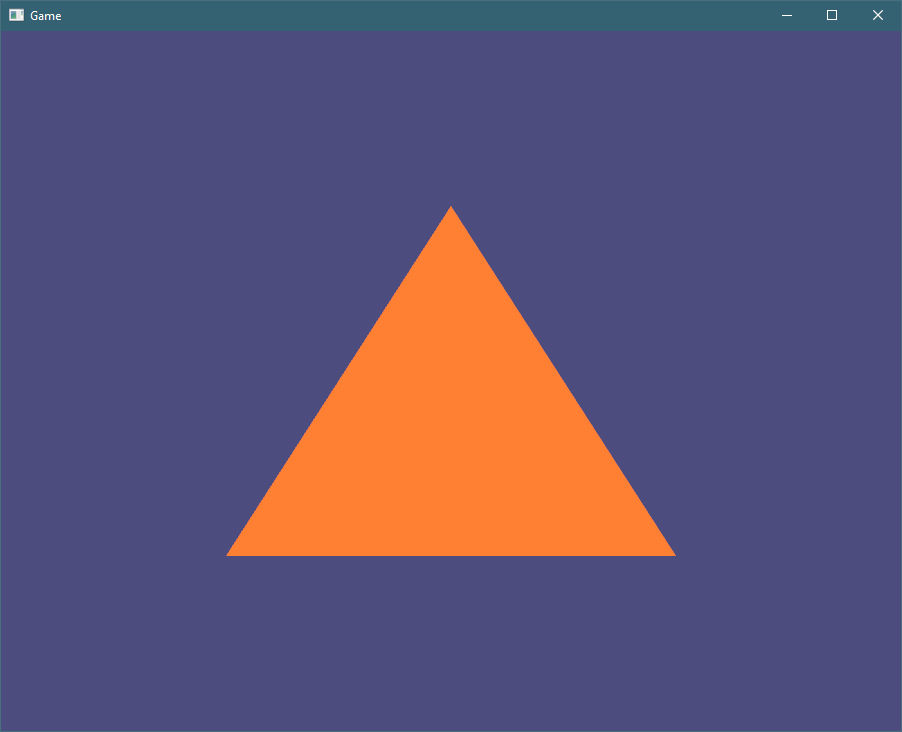Welcome back!

Previously, we have compiled some shaders and linked a shader program, and created some nice safe abstractions for both.

This time, we will send vertices to graphics pipeline, so that our shaders can transform them into pixels.

To avoid repeating the same things other OpenGL tutorials already teach, we are following learnopengl.com lessons, and we are now at Hello Triangle page.

## Sending triangle data to graphics driver

Before running the `'main` loop, initialize vector with vertices for our triangle:

(main.rs, before main loop)

``````let vertices: Vec<f32> = vec![
-0.5, -0.5, 0.0,
0.5, -0.5, 0.0,
0.0, 0.5, 0.0
];

// continue here
``````

Then, request OpenGL to give us one buffer name (as integer), and write it into Vertex Buffer Object (`vbo`) variable:

(main.rs, continued)

``````let mut vbo: gl::types::GLuint = 0;
unsafe {
gl::GenBuffers(1, &mut vbo);
}

// continue here
``````

For `GenBuffers`, we have to provide a pointer to array which it will overwrite with a new value. Rust references (`&mut` and `&`) are pointers, so we can simply pass them along. We must, of course, limit the number of buffers to `1` so it does not overwrite unknown memory nearby. That would be bad. This `unsafe` block means something.

Then, we can upload data to buffer by binding it and calling `gl::BufferData`, very much like in C:

(main.rs, continued)

``````unsafe {
gl::BindBuffer(gl::ARRAY_BUFFER, vbo);
gl::BufferData(
gl::ARRAY_BUFFER, // target
(vertices.len() * std::mem::size_of::<f32>()) as gl::types::GLsizeiptr, // size of data in bytes
vertices.as_ptr() as *const gl::types::GLvoid, // pointer to data
gl::STATIC_DRAW, // usage
);
gl::BindBuffer(gl::ARRAY_BUFFER, 0); // unbind the buffer
}

// continue here
``````

Let’s go over `BufferData` arguments:

• Target specifies buffer type, pretty much like in C
• We obtain size of data in bytes by taking size of `f32` (`std::mem::size_of::<f32>()`), multiplying it by number of items in vector (`vertices.len()`), and then casting it to whatever integer is `gl::types::GLsizeiptr`, because Rust requires explicit integer casts.
• We get pointer void pointer to data by using `.as_ptr()` function, but it would return us correct `*const f32` pointer, while OpenGL needs `*const GLvoid`. We perform explicit cast here.
• We set `gl::STATIC_DRAW` usage hint, pretty much like in C

I suggest docs.gl site for OpenGL documentation. For example, glBufferData function page contains comprehensive documentation with examples and information about supported OpenGL versions. Very useful.

At the end we unbind the buffer with `glBindBuffer(GL_ARRAY_BUFFER, 0)` call. This will make it easier to learn what needs to be bound to perform various actions.

## Data layout

To tell OpenGL about the data in our `vertices`, we need to create Vertex Array Object (VAO).

It is going to describe how to interpret the data in `vertices` and convert it to inputs for our vertex shader. In our case, our vertex shader has a single input, with an attribute location set to 0 (this is what `location = 0` does). It expects `vec3`, which is 3 `f32` values in a sequence.

First, create the VAO:

(main.rs, before loop, continued)

``````let mut vao: gl::types::GLuint = 0;
unsafe {
gl::GenVertexArrays(1, &mut vao);
}

// continue here
``````

Then, make it current by binding it:

(continued)

``````unsafe {
gl::BindVertexArray(vao);

// continue here
}
``````

To configure the relation between VAO and VBO, we also need to bind the VBO. Later, when we draw the triangle, we will only need to bind the VAO. This re-binding of VBO may be wasteful, but it makes it clear that we actually need VBO at at this step:

``````    gl::BindBuffer(gl::ARRAY_BUFFER, vbo);

// continue here
``````

And then specify the data layout for attribute 0:

(continued)

``````    gl::EnableVertexAttribArray(0); // this is "layout (location = 0)" in vertex shader
gl::VertexAttribPointer(
0, // index of the generic vertex attribute ("layout (location = 0)")
3, // the number of components per generic vertex attribute
gl::FLOAT, // data type
gl::FALSE, // normalized (int-to-float conversion)
(3 * std::mem::size_of::<f32>()) as gl::types::GLint, // stride (byte offset between consecutive attributes)
std::ptr::null() // offset of the first component
);

// continue here
``````

And then unbind both VBO and VAO, for the reasons explained earlier:

(continued)

``````    gl::BindBuffer(gl::ARRAY_BUFFER, 0);
gl::BindVertexArray(0);
``````

## Drawing

To draw our 3 vertices, we need to switch to our shader, bind the VAO, and issue the draw call:

(main.rs, in loop)

``````shader_program.set_used();
unsafe {
gl::BindVertexArray(vao);
gl::DrawArrays(
gl::TRIANGLES, // mode
0, // starting index in the enabled arrays
3 // number of indices to be rendered
);
}
``````

And if everything went smoothly, we should finally see the result:But if you have seen “classic” OpenGL tutorial traingle, you might rememeber each corner to have different color. This will be the subject of the next post.